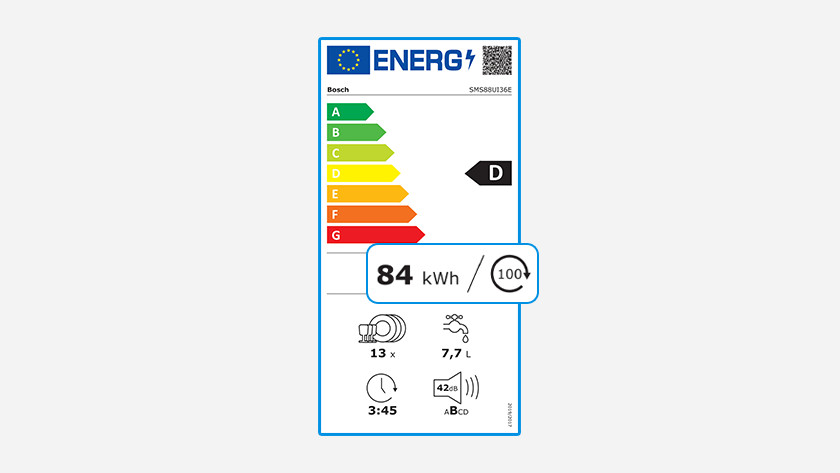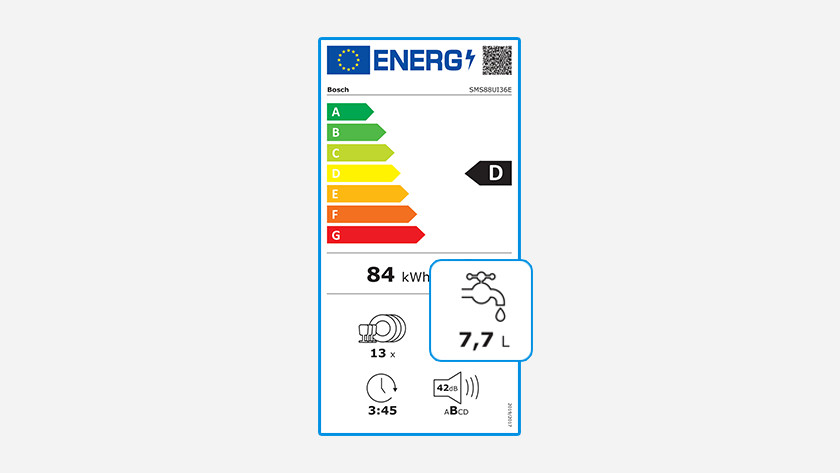Written by Rowan

# How much water and energy does a dishwasher use?

When you buy a new dishwasher, you want to know if the dishwasher is energy-efficient and if it'll save you money. The most energy-efficient dishwasher has a C energy label. in this article, we'll explain what the energy consumption of a dishwasher is and how much it'll cost you on annual energy costs on average.

## Table

Energy consumption per cycle Annual energy consumption Annual energy costs
C 0.57kWh 160kWh € 36.80
D 0.65kWh 182kWh € 41.86
E 0.73kWh 203kWh € 46.69
F 0.80kWh 225kWh € 51.75
G 0.89kWh 250kWh € 57.50

## Energy consumptionOn the energy label of a dishwasher, you can see its exact energy label and energy consumption. The most energy-efficient dishwasher has a C label. The energy costs in the table in this article are based on 280 cycles per year (over 5 cycles per week) and an energy price of € 0.23 per kWh.

## Water consumptionIf you want to know the water consumption of a dishwasher, have a look at the number below the faucet on the energy label. This is the water consumption in liters per cycle for the eco cycle. Multiply the water consumption in liters per cycle by € 0.0014. This is the average water price per liter. To calculate your annual costs, you multiply the average price by 280. For example, the energy label indicates that the water consumption is 7.7 liters per cycle. That means you'll pay an average of € 3.02 per year on water.

## Formula energy costsDo you want to calculate the average annual energy costs in euros? First, you'll have to know the yearly energy consumption. The energy label indicates the consumption per 100 cycles. In order to get the annual energy consumption, you multiply the number on the energy label by 2.8. The EU uses an estimate of 280 cycles per year. Now the sum goes € 0.23 x average energy consumption in kWh per year. If the annual energy costs are low, you save more money and you're doing the environment a favor.